图像处理 色阶_计算色阶 opencv 图像处理 - CSDN
• 阅读提示： 《C++图像处理》系列以代码清晰，可读性为主，全部使用C++代码。 《Delphi图像处理》系列以效率为侧重点，一般代码为PASCAL，核心代码采用BASM。 尽可能保持二者内容一致，可相互对照。 本文代码必须...
阅读提示：    《C++图像处理》系列以代码清晰，可读性为主，全部使用C++代码。    《Delphi图像处理》系列以效率为侧重点，一般代码为PASCAL，核心代码采用BASM。    尽可能保持二者内容一致，可相互对照。    本文代码必须包括《C++图像处理 -- 数据类型及公用函数》文章中的BmpData.h头文件。    在Photoshop中，图像色阶调整应用很广泛，本文介绍的图像色阶调整过程与Photoshop处理效果基本一致。    Photoshop的色阶调整分输入色阶调整和输出色阶调整，其中输入色阶调整有3个调整点，即通常所说的黑场、白场及灰场调整。    输入色阶调整的基本算法并不复杂，首先计算出白场与黑场的离差Diff，然后计算出像素各份量值与黑场的离差rgbDiff，如果rgbDiff<=0，像素各份量值等于0，否则，计算以rgbDiff与Diff的比值为底的灰场倒数的幂。用公式表示：    Diff = Highlight -Shadow    rgbDiff = RGB - Shadow    clRGB = Power(rgbDiff / Diff,  1 / Midtones)    其中Shadow为输入色阶低端数据（黑场），Highlight为输入色阶高端数据（白场）， Midtones为输入色阶中间数据（灰场），Diff为二者的离差（必须大于1），RGB为调整前的像素分量值，clRGB为调整输入色阶后的像素分量值。    输出色阶调整更简单，首先计算输出色阶白场与黑场的离差与255的比值系数，然后用输入色阶调整后的像素分量值乘上这个系数，再加上输出黑场值即可。用公式表示：    outClRGB = clRGB * (outHighlight - outShadow) / 255 + outShadow    其中，outShadow为输出黑场，outHighlight为输出白场，outClRGB为全部色阶调整后的像素分量值。    前面已经提到输入色阶黑白场的离差必须大于1，而输入色阶并没有这个限制，输出黑白场的离差可以为负数，当输出黑场与白场完全颠倒时，输出色阶调整后的图片为原图片的负片。    色阶调整涉及四个通道，即R、G、B各分量通道及整体颜色通道，如果每个通道单独调整，将是比较麻烦和耗时的，本文采用色阶表替换法，可一次性完成所有四个通道的色阶调整。    下面是图像色阶调整的代码：// 色阶项结构
typedef struct
{
FLOAT Midtones;
UINT Highlight;
UINT OutHighlight;
}ColorLevelItem, *PColorLevelItem;

typedef struct
{
ColorLevelItem Blue;
ColorLevelItem Green;
ColorLevelItem Red;
ColorLevelItem RGB;
}ColorLevelData, *PColorLevelData;

VOID InitColorLevelData(PColorLevelData clData)
{
PColorLevelItem item = &clData->Blue;
for (INT i = 0; i < 4; i ++, item ++)
{
item->Highlight = item->OutHighlight = 255;
item->Midtones = 1.0;
}
}

BOOL GetColorLevelTable(PColorLevelItem item, LPBYTE clTable)
{
INT diff = (INT)(item->Highlight - item->Shadow);
INT outDiff = (INT)(item->OutHighlight - item->OutShadow);

if (!((item->Highlight <= 255 && diff < 255 && diff >= 2) ||
(item->OutShadow <= 255 && item->OutHighlight <= 255 && outDiff < 255) ||
(!(item->Midtones > 9.99 && item->Midtones > 0.1) && item->Midtones != 1.0)))
return FALSE;

DOUBLE coef = 255.0 / diff;
DOUBLE outCoef = outDiff / 255.0;
DOUBLE exponent = 1.0 / item->Midtones;

for (INT i = 0; i < 256; i ++)
{
INT v;
// 计算输入色阶黑白场
v = 0;
else
{
v = (INT)((clTable[i] - item->Shadow) * coef + 0.5);
if (v > 255)
v = 255;
}
// 计算输入色阶灰场
v = (INT)(pow(v / 255.0, exponent) * 255.0 + 0.5);
// 计算输出色阶
clTable[i] = (BYTE)(v * outCoef + item->OutShadow + 0.5);
}
return TRUE;
}

BOOL CheckColorLevelData(PColorLevelData clData, BYTE clTables[])
{
BOOL result = FALSE;
INT i, j;
for (i = 0; i < 3; i ++)
{
for (j = 0; j < 256; j ++)
clTables[i][j] = (BYTE)j;
}
PColorLevelItem item = &clData->Blue;
for (i = 0; i < 3; i ++, item ++)
{
if (GetColorLevelTable(item, clTables[i]))
result = TRUE;
}
for (i = 0; i < 3; i ++)
{
if (!GetColorLevelTable(item, clTables[i]))
break;
result = TRUE;
}
return result;
}

// 图像数据色阶调整
VOID ImageColorLevel(BitmapData *dest, BitmapData *source, PColorLevelData clData)
{
UINT width, height;
INT dstOffset, srcOffset;
GetDataCopyParams(dest, source, width, height, pd, ps, dstOffset, srcOffset);

BYTE clTables;
if (CheckColorLevelData(clData, clTables))
{
for (UINT y = 0; y < height; y ++, ps += srcOffset, pd += dstOffset)
{
for (UINT x = 0; x < width; x ++, ps ++, pd ++)
{
pd->Blue = clTables[ps->Blue];
pd->Green = clTables[ps->Green];
pd->Red = clTables[ps->Red];
pd->Alpha = ps->Alpha;
}
}
}
else if (dest != source)
{
for (UINT y = 0; y < height; y ++, ps += srcOffset, pd += dstOffset)
{
for (UINT x = 0; x < width; x ++, ps ++, pd ++)
{
pd->Color = ps->Color;
}
}
}
}
    下面给一个简单的图像色阶调整函数调用例子：void __fastcall TForm1::Button1Click(TObject *Sender)
{
BitmapData dest, source;

Bitmap *sBmp = new Bitmap(L"..\\..\\media\\source1.jpg");
LockBitmap(sBmp, &source);

Bitmap *dBmp = new Bitmap(source.Width, source.Height, PixelFormat32bppARGB);
LockBitmap(dBmp, &dest);

ColorLevelData clData;
InitColorLevelData(&clData);

clData.RGB.Midtones = 1.2;
clData.RGB.Highlight = 240;
clData.RGB.OutHighlight = 200;

/*
clData.RGB.OutHighlight = 0;
*/
ImageColorLevel(&dest, &source, &clData);

UnlockBitmap(dBmp, &dest);
UnlockBitmap(sBmp, &source);

Gdiplus::Graphics g(Canvas->Handle);
g.DrawImage(sBmp, 0, 0);
g.DrawImage(dBmp, source.Width, 0);

delete dBmp;
delete sBmp;
}    下面是文章《Delphi图像处理 -- 图像色阶调整》例子运行界面效果图，第一张效果图绿色通道色阶调整，第二张效果图是RGB输出色阶调整到完全颠倒时的负片图，详细的图像色阶调整界面例子请参考《Delphi图像处理 -- 图像色阶调整》。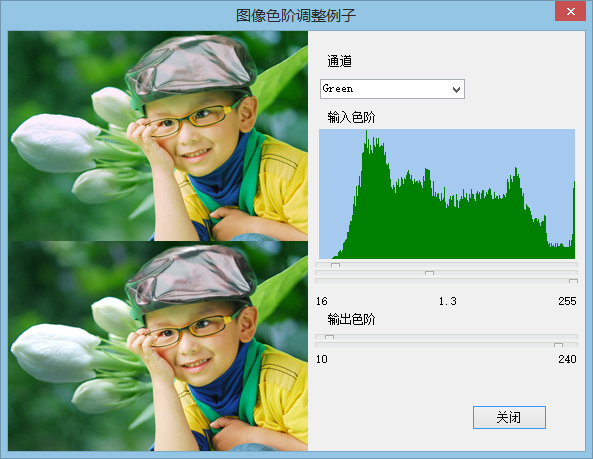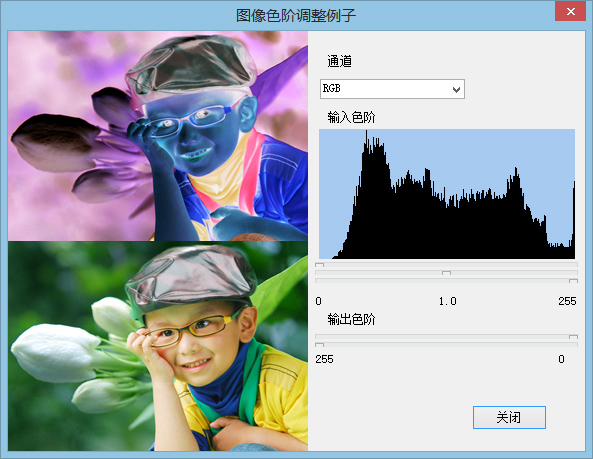本文代码系用BCB XE7编辑和编译。     因水平有限，错误在所难免，欢迎指正和指导。邮箱地址：maozefa@hotmail.com    这里可访问《C++图像处理 -- 文章索引》。﻿﻿
展开全文图像处理
• 前言：之前在公司做项目的用到photoshop颜色空间的一些相关方法，在此总结一下。下面原理部分是从我的总结文档里截取来的。需要复制的童鞋自己手写一下~ 2、程序部分 1）Matlab实验程序。...R=doub
前言：之前在公司做项目的用到photoshop颜色空间的一些相关方法，在此总结一下。下面原理部分是从我的总结文档里截取来的。需要复制的童鞋自己手写一下~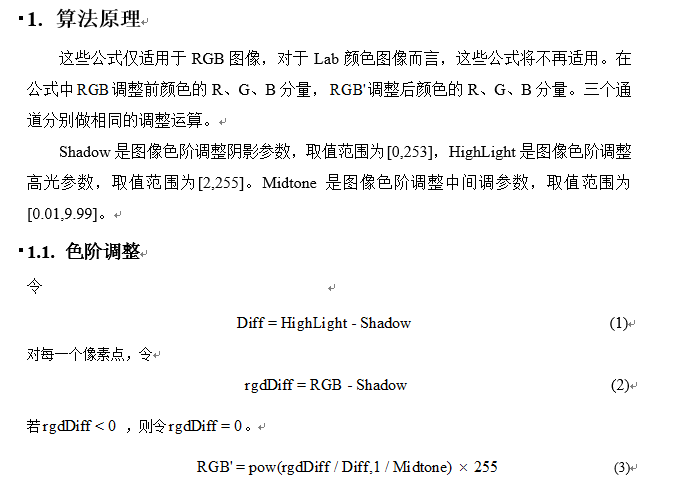2、程序部分

1）Matlab实验程序。
clc;clear;close all;
figure(1);
imshow(Image);

R=double(Image(:,:,1));
G=double(Image(:,:,2));
B=double(Image(:,:,3));

%调整参数
HighLight=238;
Midtones=0.51;

rDiff(rDiff<0)=0;
gDiff(gDiff<0)=0;
bDiff(bDiff<0)=0;

R=(rDiff/Diff).^(1/Midtones)*255;
G=(gDiff/Diff).^(1/Midtones)*255;
B=(bDiff/Diff).^(1/Midtones)*255;

R(R>255)=255;
G(G>255)=255;
B(B>255)=255;

img(:,:,1)=uint8(R);
img(:,:,2)=uint8(G);
img(:,:,3)=uint8(B);
figure(2);
imshow(img);
</span>2）C程序
void LevelAdjustRGB(unsigned char *pSrc, unsigned char *pDest, int nWidth, int nHeight,int nShadow,int nHighLight,double dMidtone)
{
//局部变量声明
int i = 0;
int nLength  = nWidth * nHeight;
int *nRgbDiff = new int[3 * nLength];

for(i = 0;i < 3 * nLength; i++)
{
if(nRgbDiff[i] < 0)
{
nRgbDiff[i] = 0;
}

//此处必须用int作为中间变量 不能用char  会有数据截断
int nTemp = static_cast<int>(pow((nRgbDiff[i] / dDiff),1 / dMidtone) * 255);
pDest[i] = CLIP8(nTemp);
}
//释放空间
if(!nRgbDiff)
{
delete []nRgbDiff;
nRgbDiff = NULL;
}
}</span>
其中#define  CLIP8(a)  (((a) & 0xFFFFFF00) ? (((a) < 0) ? 0 : 255 ) : (a))      ///<判断8位数据范围
3、实验结果，同photoshop处理结果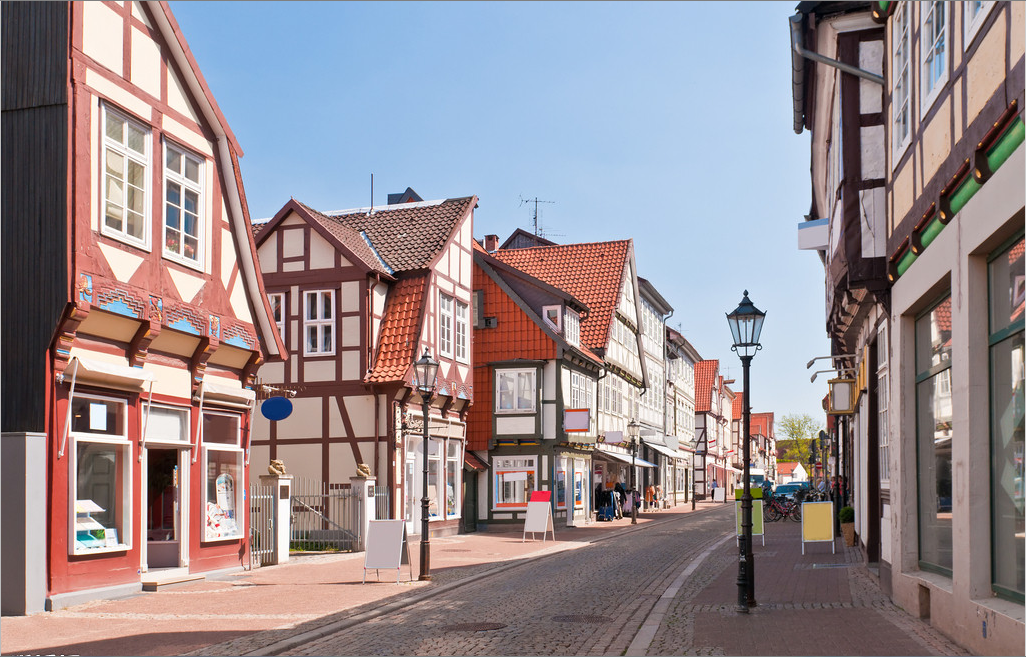图1  原图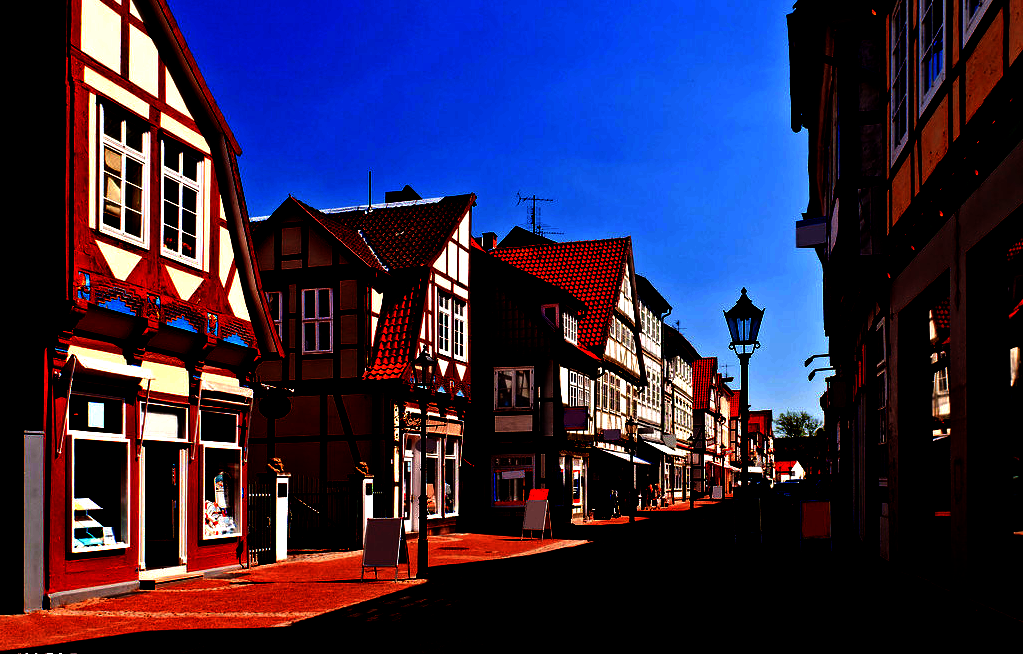nHighLight=238  dMidtone=0.51结果

展开全文photoshop 图像处理 算法 颜色空间
• 文章目录一、色阶调整( Levels Adjustment )原理二、自动色阶图像处理算法 一、色阶调整( Levels Adjustment )原理 色阶：就是用直方图描述出的整张图片的明暗信息。如图 从左至右是从暗到亮的像素分布， 黑色...
文章目录一、色阶调整( Levels Adjustment )原理二、自动色阶图像处理算法
色阶：就是用直方图描述出的整张图片的明暗信息。如图
从左至右是从暗到亮的像素分布，
黑色三角代表最暗地方（纯黑—黑点值为０），
白色三角代表最亮地方（纯白—白点为 255）。
灰色三角代表中间调。（灰点为1.00）
对于一个RGB图像, 可以对R, G, B 通道进行独立的色阶调整，即对三个通道分别使用三个色阶定义值。还可以再对 三个通道进行整体色阶调整。
因此，对一个图像，可以用四次色阶调整。最终的结果，是四次调整后合并产生的结果。
cv2.equalizeHist(img) 函数
img = cv2.imread('./20181106194742.png',0)
equ = cv2.equalizeHist(img) # 只能传入灰度图

res = np.hstack((img,equ))  # 图像列拼接（用于显示）

cv2.imshow('res',res)
cv2.waitKey(0)
cv2.destroyAllWindows()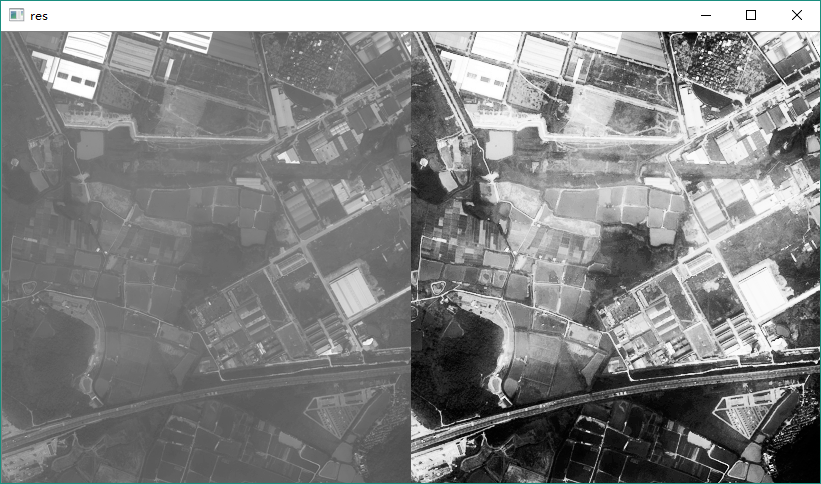二、自动色阶图像处理算法
cv2.createCLAHE() 对比度有限自适应直方图均衡
直方图均衡后背景对比度有所改善。但导致亮度过高，我们丢失了大部分信息。这是因为它的直方图并不局限于特定区域。
因此，为了解决这个问题，使用自适应直方图均衡。在此，图像被分成称为“图块”的小块（在OpenCV中，tileSize默认为8x8）。然后像往常一样对这些块中的每一个进行直方图均衡。所以在一个小区域内，直方图会限制在一个小区域（除非有噪音）。如果有噪音，它会被放大。为避免这种情况，应用对比度限制。如果任何直方图区间高于指定的对比度限制（在OpenCV中默认为40），则在应用直方图均衡之前，将这些像素剪切并均匀分布到其他区间。均衡后，为了去除图块边框中的瑕疵，应用双线性插值。
import numpy as np
import cv2

clahe = cv2.createCLAHE(clipLimit=2.0, tileGridSize=(8,8))
cl1 = clahe.apply(img)

cv2.imshow('img',img)
cv2.imshow('cl1',cl1)
cv2.waitKey(0)
cv2.destroyAllWindows()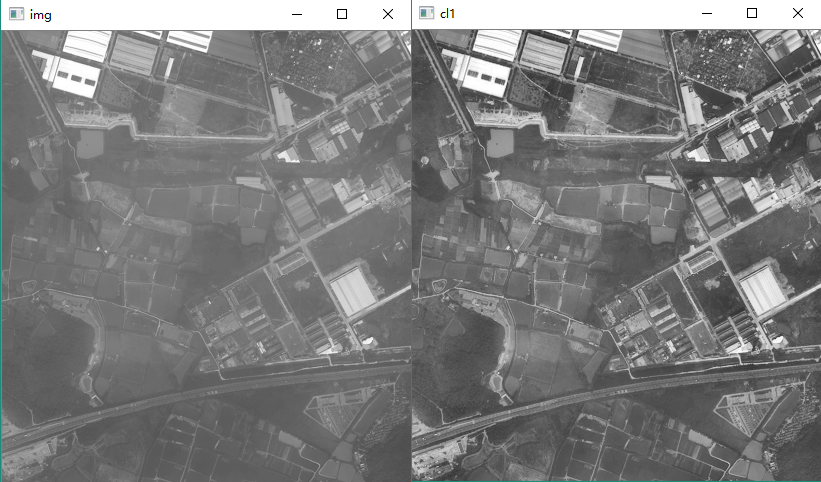自适应色阶去雾气【参看代码请点击】 主要使用numpy优化了计算耗时。
import numpy as np
import cv2

def ComputeMinLevel(hist, pnum):
return np.argwhere(index>pnum * 8.3 * 0.01)

def ComputeMaxLevel(hist, pnum):
hist_0 = hist[::-1]
index = np.argwhere(Iter_sum > (pnum * 2.2 * 0.01))
return 255-index

def LinearMap(minlevel, maxlevel):
if (minlevel >= maxlevel):
return []
else:
index = np.array(list(range(256)))
screenNum = np.where(index<minlevel,0,index)
screenNum = np.where(screenNum> maxlevel,255,screenNum)
for i in range(len(screenNum)):
if screenNum[i]> 0 and screenNum[i] < 255:
screenNum[i] = (i - minlevel) / (maxlevel - minlevel) * 255
return screenNum

def CreateNewImg(img):
h, w, d = img.shape
newimg = np.zeros([h, w, d])
for i in range(d):
imghist = np.bincount(img[:, :, i].reshape(1, -1))
minlevel = ComputeMinLevel(imghist,  h * w)
maxlevel = ComputeMaxLevel(imghist, h * w)
screenNum = LinearMap(minlevel, maxlevel)
if (screenNum.size == 0):
continue
for j in range(h):
newimg[j, :, i] = screenNum[img[j, :, i]]
return newimg

if __name__ == '__main__':
newimg = CreateNewImg(img)
cv2.imshow('original_img', img)
cv2.imshow('new_img', newimg / 255)
cv2.waitKey(0)
cv2.destroyAllWindows()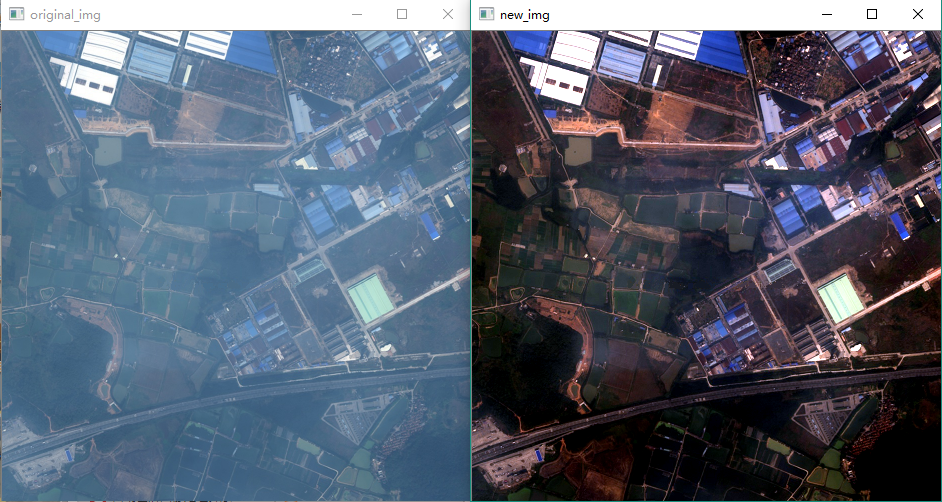展开全文• 自动色阶算法python实现
通过仿真自动色阶算法，发现其去雾效果十分明显，并且速度快于暗通道算法。
python实现：
#!python3.6

import numpy as np
import cv2

def ComputeHist(img):
h,w = img.shape
hist, bin_edge = np.histogram(img.reshape(1,w*h), bins=list(range(257)))
return hist

def ComputeMinLevel(hist, rate, pnum):
sum = 0
for i in range(256):
sum += hist[i]
if (sum >= (pnum * rate * 0.01)):
return i

def ComputeMaxLevel(hist, rate, pnum):
sum = 0
for i in range(256):
sum += hist[255-i]
if (sum >= (pnum * rate * 0.01)):
return 255-i

def LinearMap(minlevel, maxlevel):
if (minlevel >= maxlevel):
return []
else:
newmap = np.zeros(256)
for i in range(256):
if (i < minlevel):
newmap[i] = 0
elif (i > maxlevel):
newmap[i] = 255
else:
newmap[i] = (i-minlevel)/(maxlevel-minlevel) * 255
return newmap

def CreateNewImg(img):
h,w,d = img.shape
newimg = np.zeros([h,w,d])
for i in range(d):
imgmin = np.min(img[:,:,i])
imgmax = np.max(img[:,:,i])
imghist = ComputeHist(img[:,:,i])
minlevel = ComputeMinLevel(imghist, 8.3, h*w)
maxlevel = ComputeMaxLevel(imghist, 2.2, h*w)
newmap = LinearMap(minlevel,maxlevel)
# print(minlevel, maxlevel)
if (newmap.size ==0 ):
continue
for j in range(h):
newimg[j,:,i] = newmap[img[j,:, i]]
return newimg

if __name__ == '__main__':
newimg = CreateNewImg(img)
cv2.namedWindow('img',0)
cv2.imshow('img', img)
cv2.namedWindow('newimg',0)
cv2.imshow('newimg', newimg/255)
cv2.waitKey(0)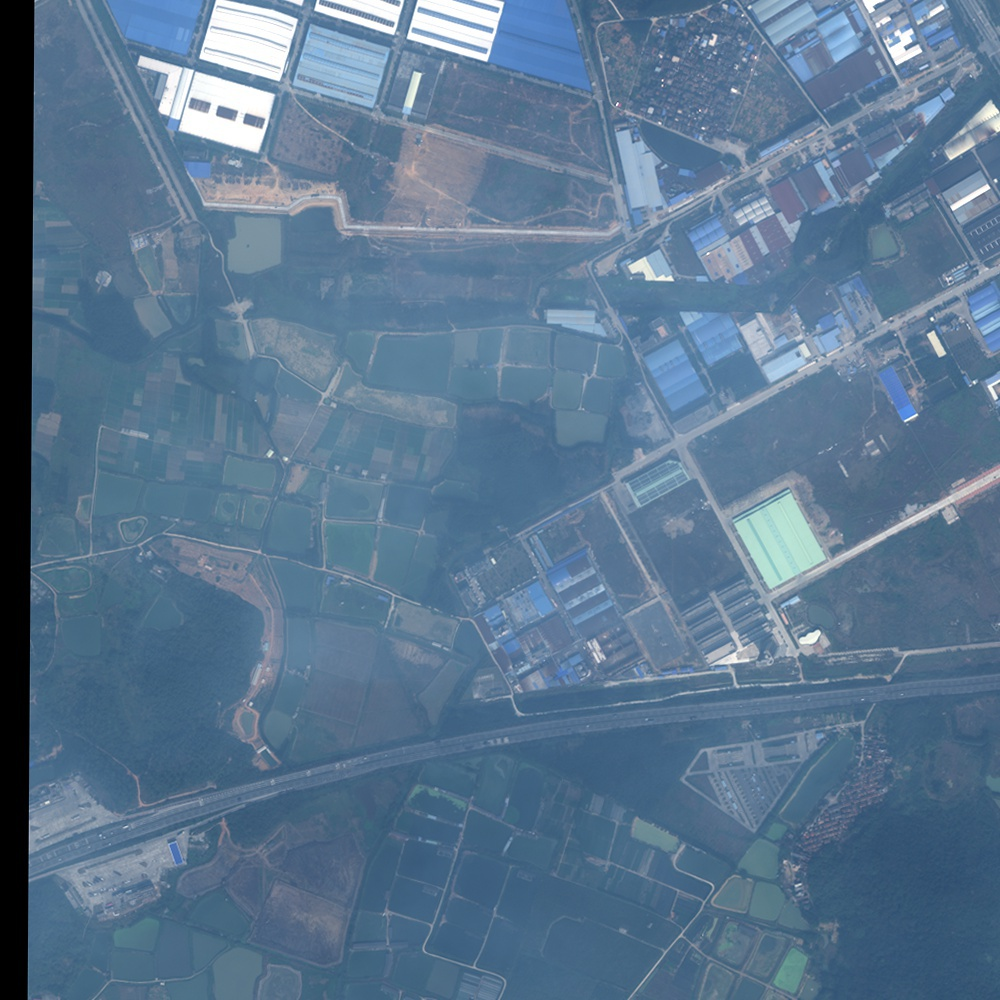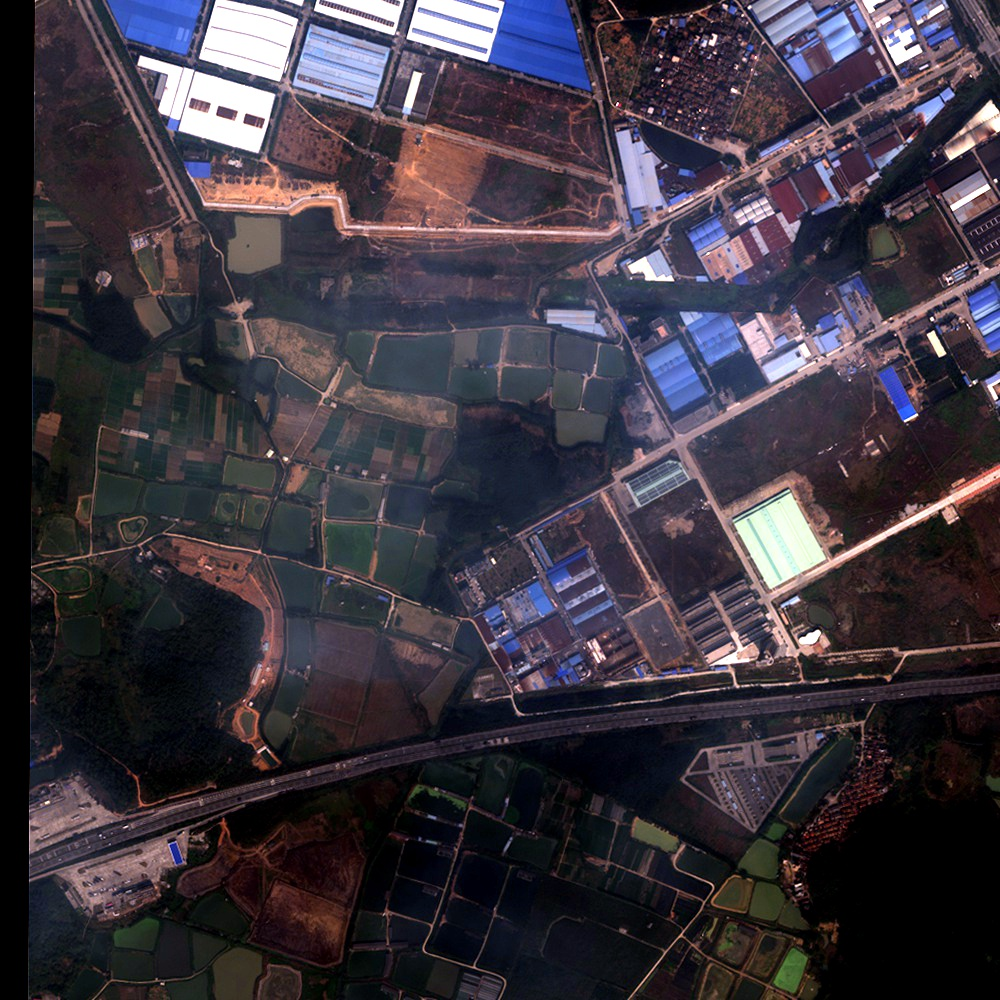展开全文图像处理 python
• import cv2 import numpy as np green=np.uint8([[[0,255,0]]]) hsv_green=cv2.cvtColor(green,cv2.COLOR_BGR2HSV) print(hsv_green) hsv_green = hsv_green print(hsv_green) img = cv2.imread("img/gre...
import cv2
import numpy as np

green=np.uint8([[[0,255,0]]])
hsv_green=cv2.cvtColor(green,cv2.COLOR_BGR2HSV)
print(hsv_green)
hsv_green = hsv_green
print(hsv_green)

#转换到HSV
hsv = cv2.cvtColor(img,cv2.COLOR_BGR2HSV)

#设定绿色的阀值
lower_green = np.array([hsv_green-100,100,100])
upper_green = np.array([hsv_green+100,255,255])
print(lower_green)
print(upper_green)
#根据阀值构建掩模
#对原图和掩模进行位运算
#显示图像
cv2.imshow('frame',img)
cv2.imshow('res',res)
cv2.imwrite("green_result.jpg",res)
cv2.waitKey(0)

原图：掩模图：结果图：参考资料链接：
Opencv-Python中文教程之颜色空间转换


展开全文• 在Photoshop中，图像色阶调整应用很广泛，本文介绍的图像色阶调整过程与Photoshop处理效果基本一致。delphi 图像处理
• OpenCV图像处理基础——基于C++实现版本视频培训课程概况：教程中会讲解到OpenCV的基础知识及使用方法，并基于OpenCV实现基础的图像处理算法；除此之外课程包含如下的内容： 图像颜色空间及类型转换及应用（BGR...计算机视觉 计算机视觉与图像处理 计算机视觉的研究方向 计算机视觉需要学什么
• VC编程实现对位图图像自动色阶处理 VC编程实现位图拷贝、切除空白边介绍了VC实现位图图像拷贝，切除二值图空白边，本文继续介绍位图处理类CImageUtility的其它成员方法，着重介绍VC编程实现位图图像自动色阶的...
• 1.自动色阶调整算法原理：（1）分别统计每个通道的灰度直方图;（2）对每个通道,利用LowCut和HighCut，计算灰度最小值min和最大值max;（3）对每个通道分别建立分段线性拉伸查找表, f(g) = 0 g&lt;=min f(g) ...
• 本篇文章讲解图像灰度化处理的知识，结合OpenCV调用cv2.cvtColor()函数实现图像灰度操作，使用像素处理方法对图像进行灰度化处理。基础性知识希望对您有所帮助。 1.图像灰度化原理 2.基于OpenCV的图像灰度化处理 3....Python 图像处理 OpenCV 灰度化处理
• 基于C#开发的图片色阶处理工具，按照PhotoShop的自动色阶10%的参数处理图片色阶。开放源代码可直接嵌入其他程序中。
• 医学图像处理的对象是各种不同成像机理的医学影像，临床广泛使用的医学成像种类主要有X-射线成像 （X-CT）、核磁共振成像（MRI）、核医学成像（NMI）和超声波成像（UI）四类。在目前的影像医疗诊断中，主要是通过...图像处理
• 自动色阶算法用于图像...如下图，在图像处理中是不是很熟悉?! 本文主要参考人在旅途的博客，采用matlab语言实现，算法不在详细叙述，在此代码留做备注。http://www.cnblogs.com/Imageshop/archive/2011/11/13/224...
• 调整图像- 自动对比度、自动色阶算法 　我们以24位彩色图像为例说明这两个算法。 　在执行两个算法之前，我们需要确定两个参数，大家在用PS时选择自动色阶并不会弹出什么参数设置对话框，那是因为PS把这个隐藏的...
• 预处理选用的是ps中的色阶处理，可是cv2等图像库中没有现成的函数，只能自己一点一点搜资料，找到了ps中色阶调整的计算公式。 以下是ps中的色阶窗口 左边黑三角叫做黑场，中间叫做灰场，右边叫做白场,上面的图是...ps 神经网络 图片预处理
• 马赛克是一种广为使用的图像处理手段，它是将影像特定区域的色阶细节劣化并造成色块打乱的效果。这种模糊看样子像一个个小格子，所以被称为马赛克。马赛克主要的目的就是使图像内容无法辨认，以保护特殊图像内容。...
...